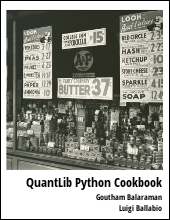Describes how to value options on commodity futures contract using the Black formula in QuantLib Python

Visit here for other QuantLib Python examples. If you found these posts useful, please take a minute by providing some feedback.

The Black-Scholes equation is the well known model to price equity European options. In the case of equities, the spot price fluctuates and hence the intuition to model as a stochastic process makes sense. In the case of commodities, however, the spot price does not fluctuate as much, and hence cannot be modeled as a stochastic process to value options on commodities. In the 1976 paper , Fischer Black addressed this problem by modeling the futures price, which demonstrates fluctuations, as the lognormal stochastic process.

The futures price at time $t$, $F_t$ with a is modeled as:

\begin{eqnarray} dF_t &=& \sigma_t F_t dW \end{eqnarray}

where $\sigma_t$ is the volatility of the underlying, and $dW$ is the Weiner process.

The value of an option at strike $K$, maturing at $T$, risk free rate $r$ with volatility $\sigma$ of the underlying is given by the closed form expression:

\begin{eqnarray} c &=& e^{-r T} [FN(d_1) - KN(d_2)] \nonumber \\ p &=& e^{-r T} [KN(-d_2) - FN(-d_1)] \end{eqnarray}

where \begin{eqnarray} d_1 &=& \frac{\ln(F/K) + (\sigma^2/2)T}{\sigma\sqrt{T}} \nonumber\\ d_2 &=& \frac{\ln(F/K) - (\sigma^2/2)T}{\sigma\sqrt{T}} = d_1 - \sigma\sqrt{T} \end{eqnarray}

This formula can be easily used to price caps, swaptions, futures options contract. Here we will use QuantLib to price the options on commodity futures.

In :
import QuantLib as ql
import math

In :
calendar = ql.UnitedStates()
bussiness_convention = ql.ModifiedFollowing
settlement_days = 0
day_count = ql.ActualActual()


## Option on Treasury Futures Contract

Options on treasury futures (10 Yr Note TYF6C 119) can be valued using the Black formula. Let us value a Call option maturing on December 24, 2015, with a strike of 119. The current futures price is 126.95, and the volatility is 11.567%. The risk free rate as of December 1, 2015 is 0.105%. Let us value this Call option as of December 1, 2015.

In :
interest_rate = 0.00105
calc_date = ql.Date(1,12,2015)
yield_curve = ql.FlatForward(calc_date,
interest_rate,
day_count,
ql.Compounded,
ql.Continuous)

In :
ql.Settings.instance().evaluationDate = calc_date
option_maturity_date = ql.Date(24,12,2015)
strike = 119
spot = 126.953# futures price
volatility = 11.567/100.
flavor = ql.Option.Call

discount = yield_curve.discount(option_maturity_date)
strikepayoff = ql.PlainVanillaPayoff(flavor, strike)
T = yield_curve.dayCounter().yearFraction(calc_date,
option_maturity_date)
stddev = volatility*math.sqrt(T)

black = ql.BlackCalculator(strikepayoff,
spot,
stddev,
discount)

In :
print "%-20s: %4.4f" %("Option Price", black.value() )
print "%-20s: %4.4f" %("Delta", black.delta(spot) )
print "%-20s: %4.4f" %("Gamma", black.gamma(spot) )
print "%-20s: %4.4f" %("Theta", black.theta(spot, T) )
print "%-20s: %4.4f" %("Vega", black.vega(T) )
print "%-20s: %4.4f" %("Rho", black.rho( T) )

Option Price        : 7.9686
Delta               : 0.9875
Gamma               : 0.0088
Theta               : -0.9356
Vega                : 1.0285
Rho                 : 7.3974


## Natural Gas Futures Option

I saw this question on quantlib users group. Thought I will add this example as well.

Call option with a 3.5 strike, spot 2.919, volatility 0.4251. The interest rate is 0.15%.

In :
interest_rate = 0.0015
calc_date = ql.Date(23,9,2015)
yield_curve = ql.FlatForward(calc_date,
interest_rate,
day_count,
ql.Compounded,
ql.Continuous)

In :
ql.Settings.instance().evaluationDate = calc_date
T = 96.12/365.

strike = 3.5
spot = 2.919
volatility = 0.4251
flavor = ql.Option.Call

discount = yield_curve.discount(T)
strikepayoff = ql.PlainVanillaPayoff(flavor, strike)
stddev = volatility*math.sqrt(T)

strikepayoff = ql.PlainVanillaPayoff(flavor, strike)
black = ql.BlackCalculator(strikepayoff, spot, stddev, discount)

In :
print "%-20s: %4.4f" %("Option Price", black.value() )
print "%-20s: %4.4f" %("Delta", black.delta(spot) )
print "%-20s: %4.4f" %("Gamma", black.gamma(spot) )
print "%-20s: %4.4f" %("Theta", black.theta(spot, T) )
print "%-20s: %4.4f" %("Vega", black.vega(T) )
print "%-20s: %4.4f" %("Rho", black.rho( T) )

Option Price        : 0.0789
Delta               : 0.2347
Gamma               : 0.4822
Theta               : -0.3711
Vega                : 0.4600
Rho                 : 0.1597


## Conclusion

In this notebook, I demonstrated how Black formula can be used to value options on commodity futures. It is worth pointing out that different vendors usually have different scaling conventions when it comes to reporting greeks. One would needs to take that into account when comparing the values shown by QuantLib with that of other vendors

 Fischer Black, The pricing of commodity contracts, Journal of Financial Economics, (3) 167-179 (1976)